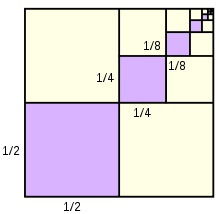# Calculate 6414

If we add the same number x to the numbers -1,3,15,51, we get the first four members of the geometric sequence. Calculate the number x and the first four members of the geometric sequence.

x =  3
a1 =  2
a2 =  6
a3 =  18
a4 =  54

### Step-by-step explanation:Did you find an error or inaccuracy? Feel free to write us. Thank you!

Tips for related online calculators
Are you looking for help with calculating roots of a quadratic equation?
Do you have a linear equation or system of equations and looking for its solution? Or do you have a quadratic equation?